# # How to Find the Longest Word in a String in JavaScript

In today's pictorial, let's learn how to get the longest word in a given string. As you solve more of these algorithm challenges, you will notice there is rarely a direct easy path solution. Often times, you will need to covert one certain data type to another, so you can access the built in methods. For example, converting a string to an array in order to access the `map` method. Don't worry the more you practice, the easier these challenges will become 🤓

Here are the 4 methods I'll be covering:## # The Challenge

Write a function that returns the length of the longest word in a sentence.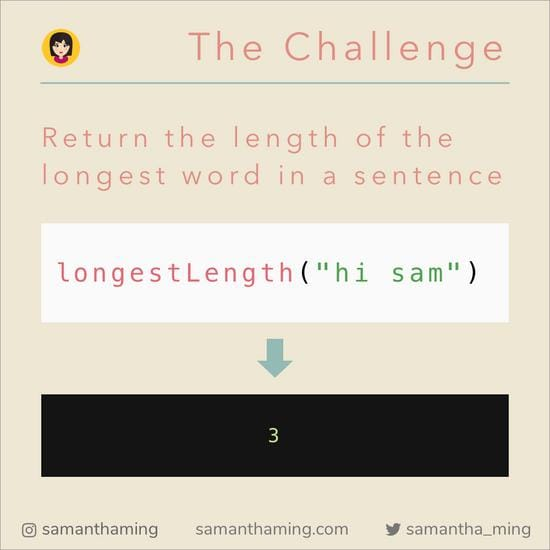## # 1. `split()`

The general direction of finding a string is to access the `length` property. However, we can't just call this on the entire string because it will just return the length of the entire sentence. So, we need to split our sentence into separate words. Then, we can collect the `length` of each word. And from the collection of lengths, we find the largest number and return the corresponding word. Clear as mud 😂 Let's just view the pictorial and this will make more sense 💪

Split our sentence into an array of words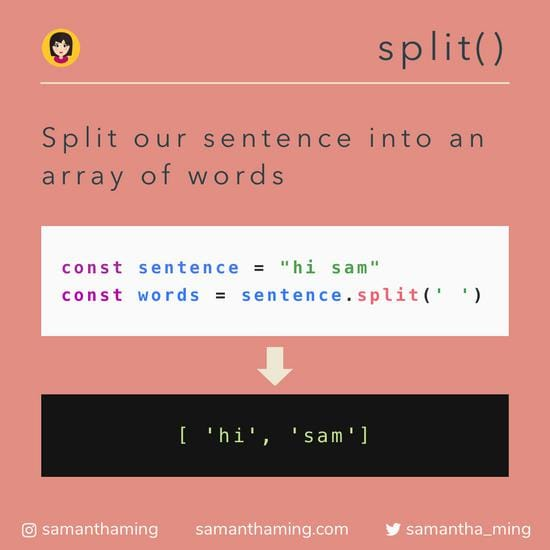## # 2. `length`

We can call the `length` property to access a string.

Retrieve the length of a string.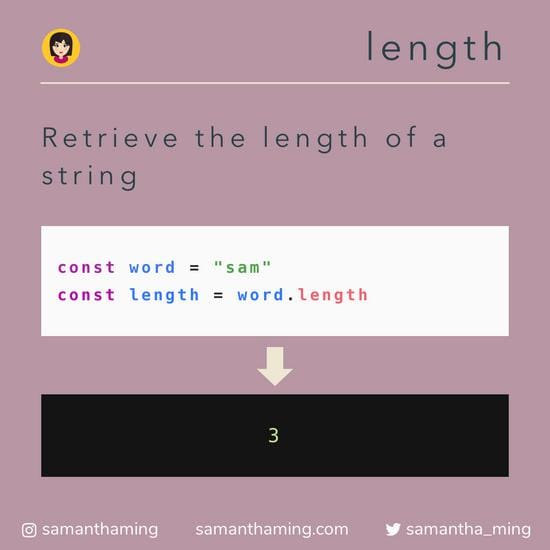## # 3. `map()`

With the `split()` method, we now have an array of words. And now we want to call the `length` property of each word while collecting the value into an array. To loop over an array while applying "some action" to each item, we can use `map()`.

Create a new array with the length of the words.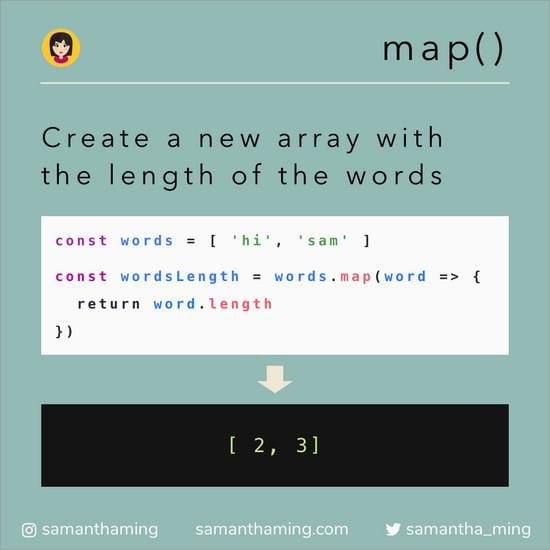## # 4. `Math.max`

Now that we have an array filled with lengths. We want to find the largest number of the bunch.

Retrieve the largest number from the array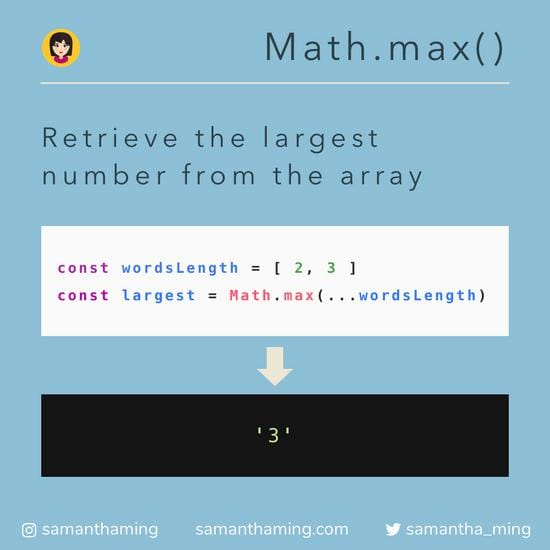## # Final Solution

Now combine all these methods together, we have successfully find the longest word in a string. Yay!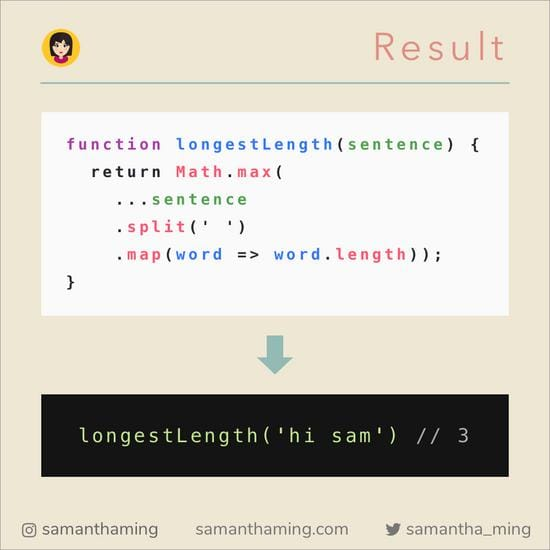## # More Solutions

``````function longestWordLength(str) {
return Math.max(...str.split(' ').map(word => word.length));
}
``````

Using 2 steps

``````function longestWordLength(str) {
const arrLength = str.split(' ').map(s => s.length);
return Math.max(...arrLength);
}
``````

Using Reduce

``````function longestWordLength(str) {
return str.split(' ').reduce((maxNumber, current) => {
return current.length > maxNumber ? current.length : maxNumber;
}, 0);
}
``````

Using Reverse

``````function longestLength(str) {
return str
.split(' ')
.map(s => s.length)
.reverse();
}
``````

Know other solutions? Submit it here